Company Analysis

# UBA Plc: Time To Boost Earnings Growth, Check Expenses, Improve Ratios

Rating: Buy
Current Market Price: N7.10

Loan to Deposit Ratio: 44.64%
Year High: N9.25
Year Low: N6.75
Fair Value: N11.26
Equity Analyst: Tunde Segun Jeariogbe

Key Investment Ratios

• This report observed the first quarter financial performance indices of United Bank for Africa (UBA) for the period ended 31st March, 2021, compared them with similar figures released in the corresponding period of 2020 to establish growth and make projections where necessary.
• We adjusted our valuation slightly below that of full-year to place traders on the safer side.
• It is important to understand that our valuation, though conservative, is more appropriate for long-term investors, (five financial years). Despite this, we expect short-term traders to play within the technical trading gap.
• Generally, the bank’s earnings stood at an average point above that of the comparable quarter, following which we commend the management for outperforming the economy.
• See below for detailed analysis of the released numbers:Sources: Company Data, NGX, investdata Research

Company figures

• Gross Earnings through the quarter stood at N155.44 billion, same as 5.62% above the N147.16 billion posted in its 2020 first quarter.
• Interest Income dipped by a marginal 0.47% to N108.59 billion, as against N109.10 billion in the corresponding quarter.
• Interest Expenses is currently valued at N34.20 billion, 21.70% below the N43.69 billion posted at the end of 2020Q1.
• Operating Expenses is estimated at N59.60 billion, higher than N54.47 billion used through the 2020 first quarter by 9.41%.
• Profit before Tax is estimated at N40.58 billion versus N32.72 billion in the comparable quarter of 2020.
• After taking care of the Tax Expense, the management of UBA reported N38.15 billion as profit for the quarter, which is 26.76% higher than the N30.10 billion earned in the preceding Q1.Sources: Company Data, NGX, investdata Research
• At the end of the 2021 first quarter, the bank’s Total Assets was valued at N7.89 trillion, same as 24.27% above the N6.35 trillion stated in its 2020 first quarter.
• Total Liabilities equally stood above comparable quarter by 24.25% to N7.12 trillion, versus N5.73 trillion at the end of 2020Q1.
• Net Assets is valued at N762.37 billion, as against N612.63 billion posted at the end of the corresponding quarter.
• Total Deposit received through the first months of 2021 is estimated at N6.16 trillion, which is 32.00% above N4.67 trillion recorded in 2020 first quarter.
• Total Loans and Advances at the end of the 2021 first quarter stood at N2.75 trillion, 19.42% higher than the N2.30 trillion posted in the similar period of 2020.
• See the above table for details:

Financial Strength/Solvency Ratios

• Debt Ratio which compares Total Assets to Total Liabilities stood at 90.34%, as against the 90.35% reported in the corresponding quarter. It is important to note that the high debt ratio is typical of financial institutions.
• Total Debt for the quarter can replicate equity in 9.35 times, as against 9.37 times in the corresponding quarter. As noted above, this is because of the deposit taking nature of the banks.
• Equity Ratio which compares Equity to Total Assets stood at 9.66%, as against 9.65% in the corresponding quarter of last year.
• Estimated Beta value for UBA as of this time this report was compiled, stood at 1.20x above market beta and industry average.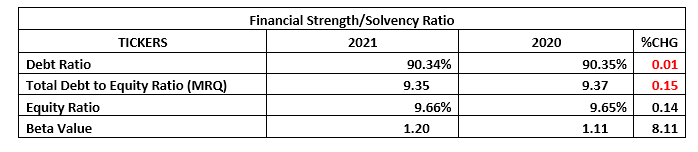Sources: Company Data, NGX, investdata Research

Profitability Ratios

• Pre-Tax Margin improved by 17.40% to stand at 26.11%, as against the 22.24% estimated at the end of 2020 first quarter.
• Interest Expenses to Gross Earnings dropped by 25.87% to 22.01% against the previously estimated 29.69% in the corresponding quarter.
• Return on Average Equity through the quarter was 5.00%, as against 4.91% in the 2020 first quarter.
• See below table for detailed profitability ratios and comparison.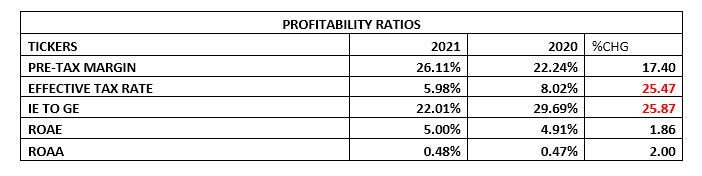Sources: Company Data, NGX, investdata Research

Efficiency Ratios

• Operating Expenses for the period is estimated as 38.35% of the Gross Earnings figure, this is only 3.59% above the 37.02% achieved in the corresponding quarter of last year.
• Gross Earnings is same as 1.97% of the Total Assets valuation at the end of the quarter, which is 15.00% below the 2.32% estimated in 2020.
• It was equally established that 44.64% of the total deposits for the period was given out as loans, 9.53% below the 49.35% deposit recorded in loan and advances through the 2020 first quarter, which is below the 65% thresholds.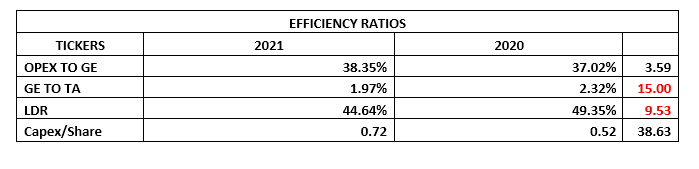Sources: Company Data, NGX, investdata Research

Investment/Valuation Ratios

• At the end of the quarter, N1.12 was earned on each share of UBA, which is higher than the N0.88 earned per share at the end of 2020 first quarter.
• Our first quarter adjusted P/E Ratio stood at 1.52x, as against 1.69x in the corresponding quarter.
• The above-mentioned EPS is same as a 16.41% yield on the Nigerian Exchange when the result was released to investors, a higher yield when compared to the 14.79% yield in the 2020 first quarter.
• Estimated Book Value per share of UBA is currently N22.29, same as 24.44% above the N17.91 estimated at the end of 2020 first quarter.
• Thus, Price to Book Value stood at 0.31x, as against the previous 0.33x, which simply implies that the stock is currently sold below its book value on the exchange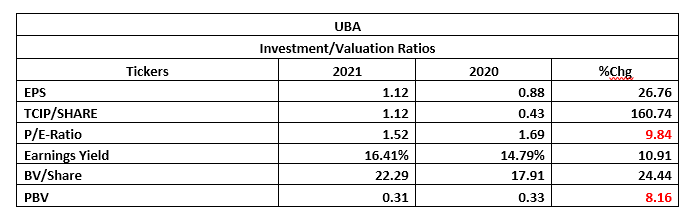Sources: Company Data, NGX, investdata Research

Valuation

While trying to place a fair value on each unit of UBA shares, we considered the average of two valuation models. FCFE Stable Growth Model valued the stock at N17.53 while H-Model placed the price at N4.99, due to lower dividend which depleted the income expectations from UBA in the number of projected years. We therefore arrived at an average value of N11.26 for each unit of its listed shares, following which we rated UBA shares a BUY.

FIVE YEARS FINANCIAL HISTORY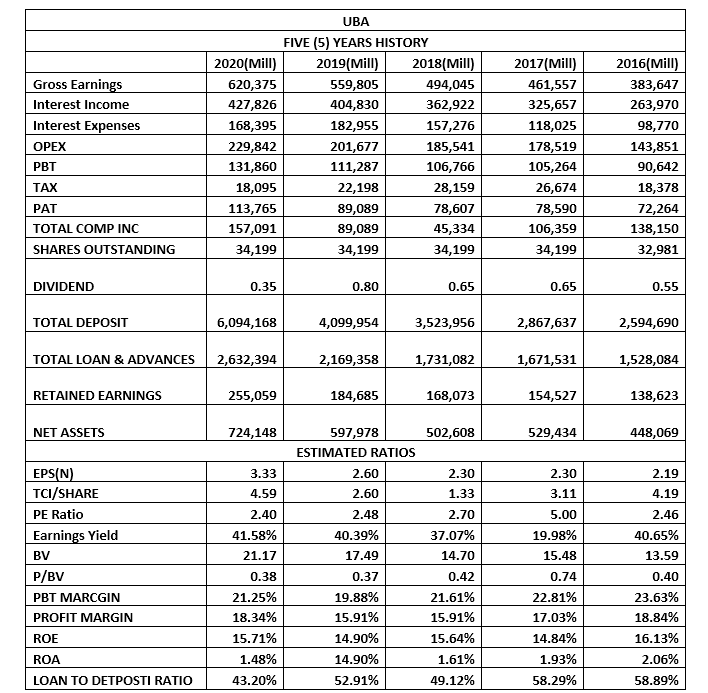Sources: Company Data, NGX, investdata Research

Check Also
Close